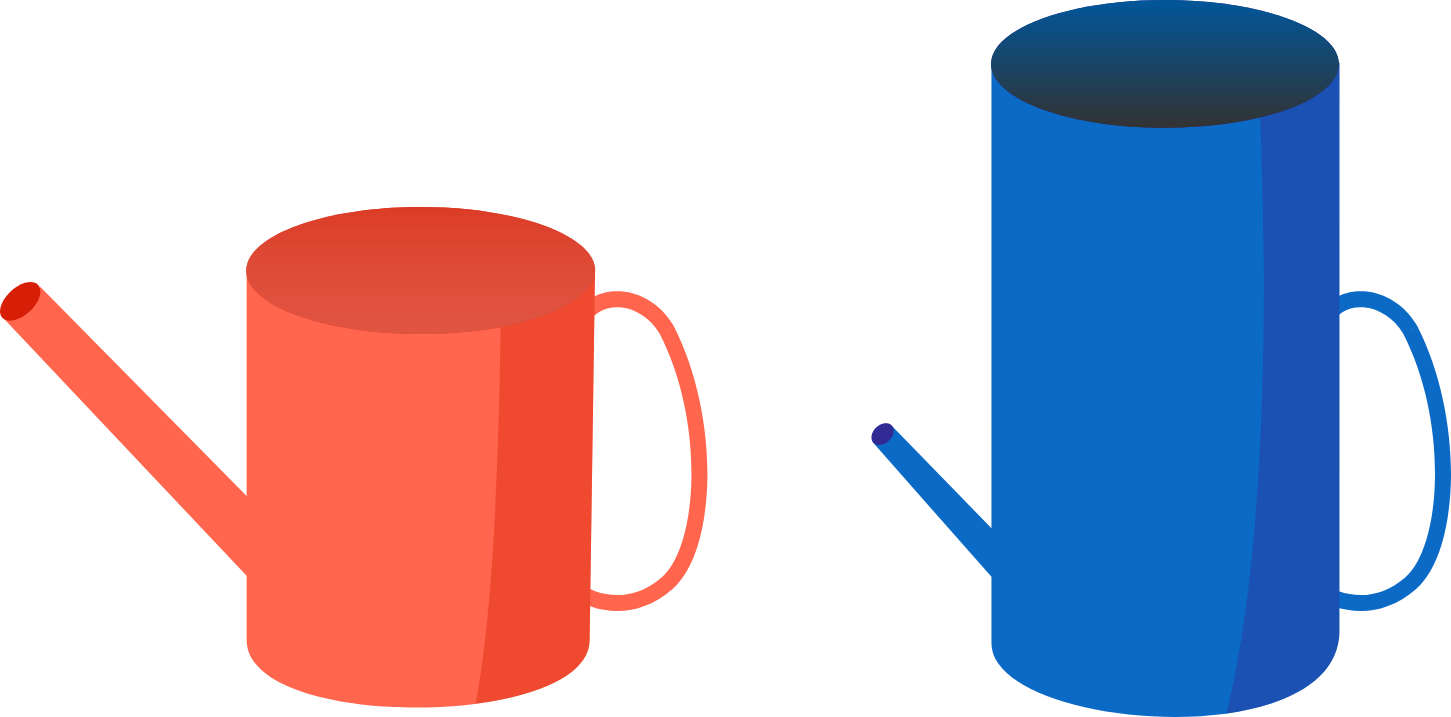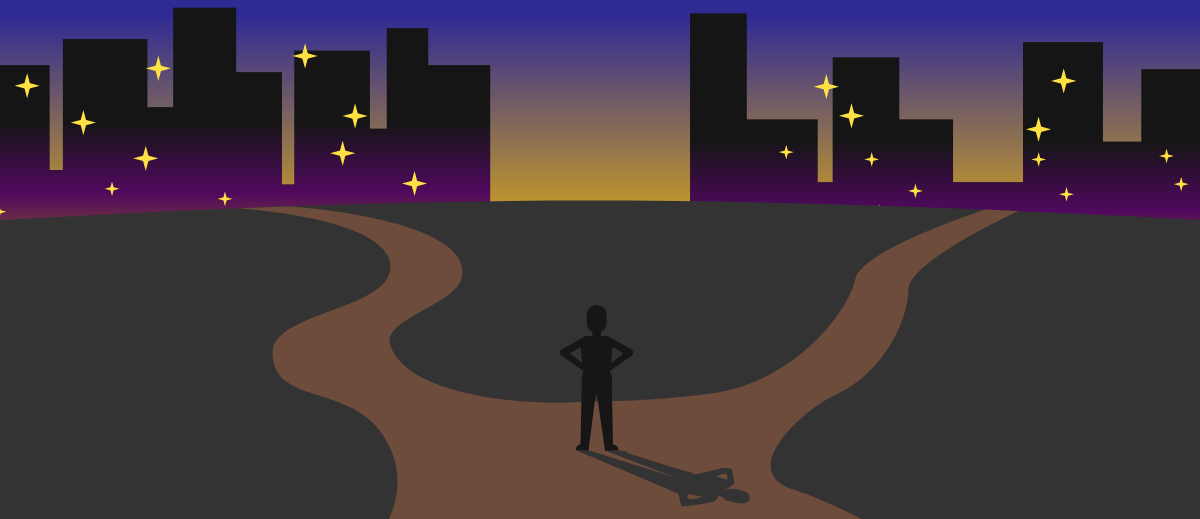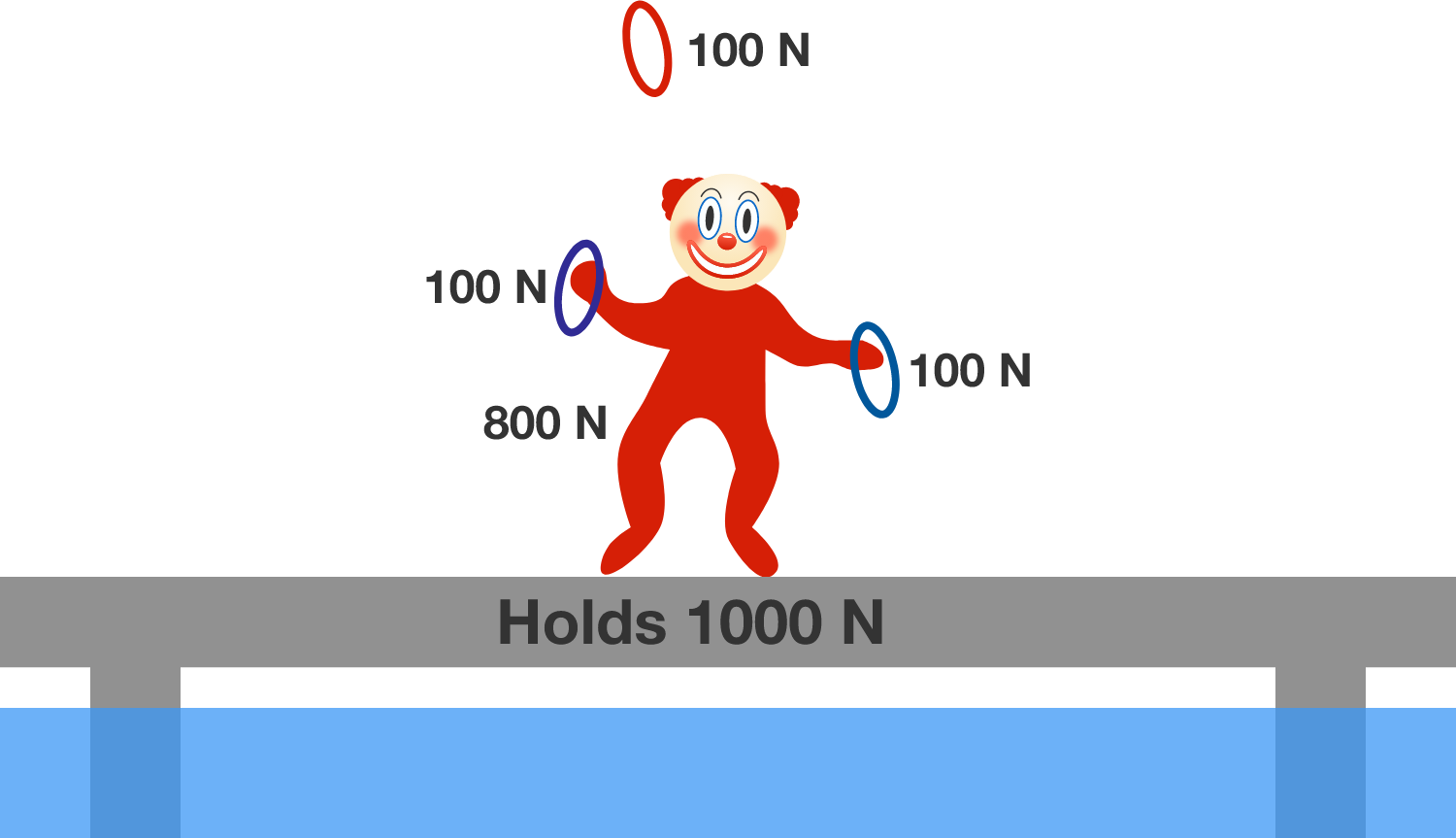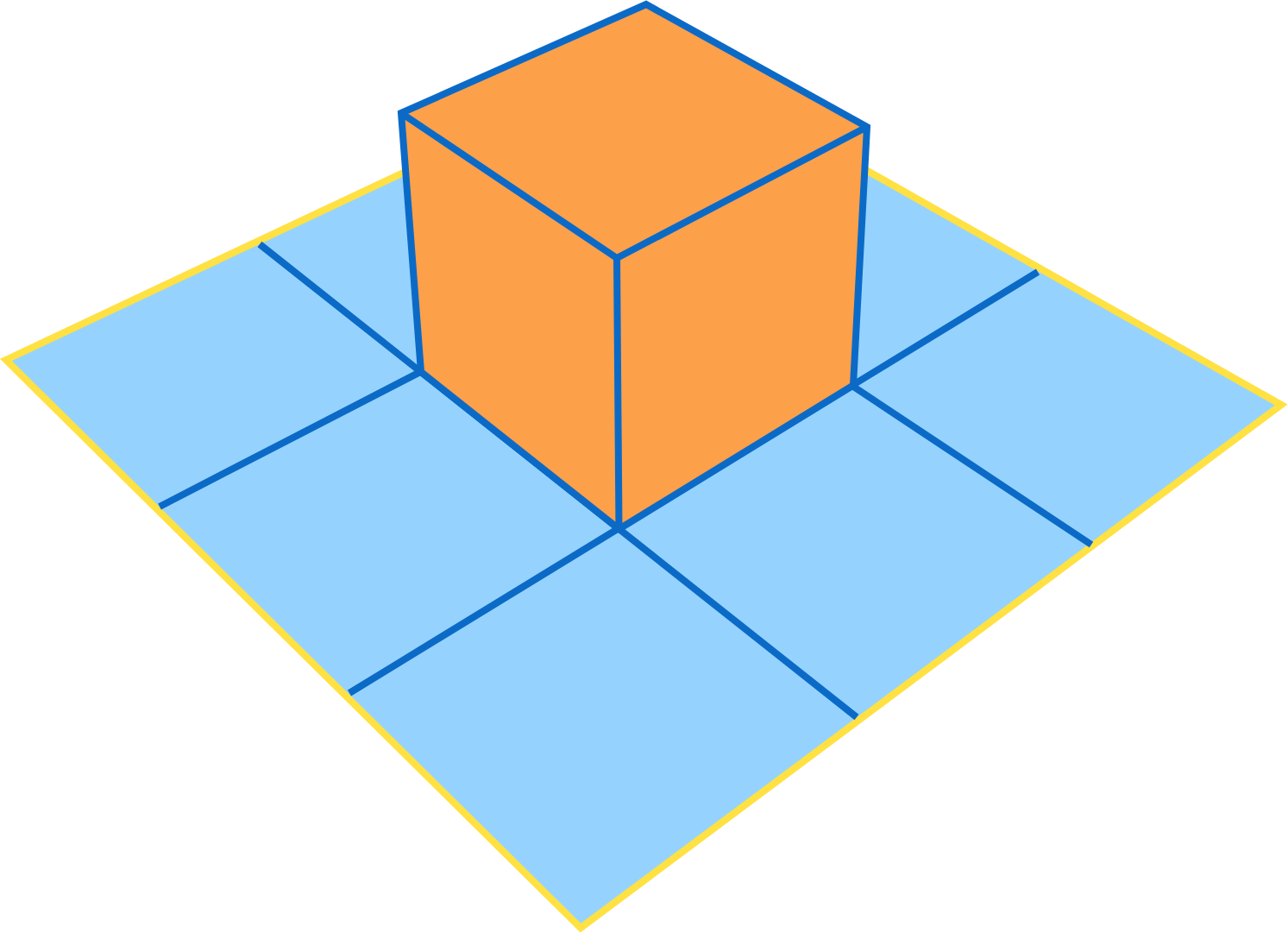# Problems of the Week

Contribute a problem

# 2017-05-15 BasicThe two watering cans above have equal base areas. Their spouts are both open. Which of the two cans can hold a greater volume of water in equilibrium in the upright positions as shown above?

You reach a fork in a road: one path leads to Truth City while the other leads to Lies City.

You encounter a man who you know is either from Truth City or Lies City. If he is from Truth City, he will tell the truth, and if he from Lies City, he will lie.

What question will make him point to Truth City?$\begin{array} { rrrrr } & & & & & A \\ & & & & A & A \\ & & & A & A & A \\ + & & A & A & A & A \\ \hline & \large\square & \large\square & \large\square & \large\square & \large\square \\ \end{array}$

The above is an incomplete cryptogram, where $A$ is a positive, single-digit integer. As shown, the final sum is a 5-digit number.

What is this final sum?

Bobo the Clown wants to cross a bridge over dangerous waters while performing a three ring juggle, in which one or two rings are in the air at all times. The bridge can support a maximum weight of $\SI[per-mode=symbol]{1000}{\newton},$ above which it will collapse. Each ring weighs $\SI[per-mode=symbol]{100}{\newton}$ and Bobo himself weighs $\SI[per-mode=symbol]{800}{\newton}.$Coco the Clown tells Bobo that it will be safe to cross the bridge as long as Bobo is never holding all three rings at once. Dodo the Clown disagrees, arguing that the juggle will collapse the bridge, even if at least one ring is in the air at all times.

Who is correct, Coco or Dodo?We have a $1\times 1\times 1$ orange cube on a piece of $3\times 3$ blue paper. Is it possible to cover the entire cube with the paper under the following conditions?

1. The paper can only be cut or folded along the grid.
2. The cut should not cause the paper to separate into pieces.
×